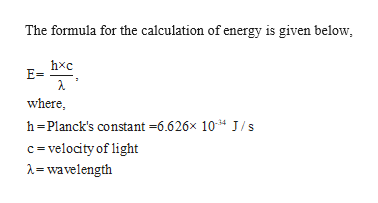Hospital X-ray generators emit X-rays with wavelength of about 15.0 nanometers (nm), where 1nm=10−9m. What is the energy of a photon of the X-rays?

Question
Hospital X-ray generators emit X-rays with wavelength of about 15.0 nanometers (nm), where 1nm=10−9m. What is the energy of a photon of the X-rays?

Step 1

Given,

Wavelength = 15.0 nm

1 nm =...help_outlineImage TranscriptioncloseThe formula for the calculation of energy is given below E= where h-Planck's constant -6.626x 1034 J/s c velocity of light =wavelength fullscreen

Want to see the full answer?

See Solution

Want to see this answer and more?

Our solutions are written by experts, many with advanced degrees, and available 24/7

See Solution
Tagged in

General Chemistry